# Amplifier

DEFINITION

An amplifier is a device that increase the voltage, current or power of an input signal with the aid of transistor by furnishing the additional power from a separate dc source.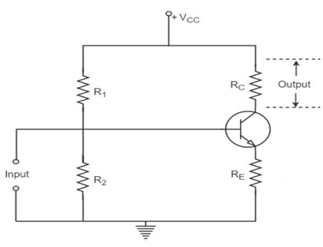COMMON EMITTER AMPLIFIER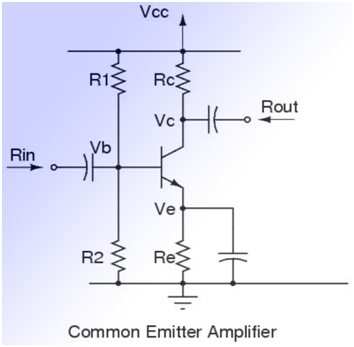The circuit arrangement of single stage common emitter amplifier. A practical amplifier uses different circuitry components to achieve faithful amplification. The functions of this amplifier circuit is discussed as:

1. Biasaing / Stabilization Circuit
2. Coupling / Blocking Capacitor (Cin/C)
3. Bypass Capacitor (CE)

a. Biasaing / Stabilization Circuit: The resistances R1, R2 and RE form the voltage divider biasing circuit for the CE amplifier. It basically sets the proper operating point for the CE amplifier. It basically sets the proper operating point for the CE amplifier.

b. Coupling / Blocking Capacitor (Cin/C): Its main function is to couple the signal to the base of the transistors. It blocks any dc component present in signal and passes ac signal only for amplification.

c. Bypass Capacitor (CE): It is connected in parallel with the emitter resisitance RE to provide a low reactance path to the amplified ac signal. It is not connected then the amplified ac signal passing through RE will cause a voltage drop across it. As a result, the output voltage get reduced and thus reducing the gain of the amplifier.

COMMON COLLECTOR AMPLIFIER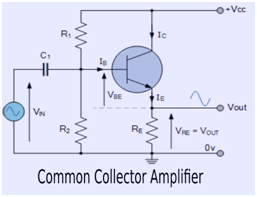The common –collector circuit where the dc-bias is provided by R1 R2 and RE. When a signal is applied via to the base of the transistor, VB increase and then decrease as the signal goes from positive and negative respectively. The voltage across the emitter resistance RE is V which is given as,

VE = VB-BE

Where, VBE is a constant.

Hence, the emitter voltage VE will vary in the same manner as the base voltage VB. Thus, the output voltage(VE) closely follows the input voltage(VB), both in phase and magnitude. Hence, its  other name is Emitter Followers. This configuration has high input impedance and hence it can be used in interfacing voltage sources with relatively high output resistance. Also, it has low output impedance, it can drive large current into load. This property is known as buffering. The use of emitter follower between source and load isolates low impedances loads from high impedance sources. This is known as impedance buffering. This configuration is also known as Unity Gain Follower due to its unity gain.

COMMON BASE AMPLIFIER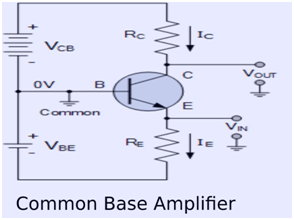The common-base amplifier circuit. The signal source is coupled to the emitter of the transistor through the coupling capacitor CC1.   The load resistance RL is connected to the collector of the transistor through the output coupling capacitor CC2. When input signal is   applied at the emitter, the positive going pulse of input source increase the emitter voltage. As base voltage is constant, the forward   bias of emitter base junction reduces. This reduces IB, resulting in reduction of IC and the voltage drop across RC. Since, the output   voltage, V0 = VCC ­– ICR, the reduction in IC results in an increase in Vo. Thus, the positive half cycle of the input produces the   positive half cycle of the output and vice versa. Therefore, there is no phase shift between input and output in common base   amplifier.

COMMON SOURCE JFET AMPLIFIER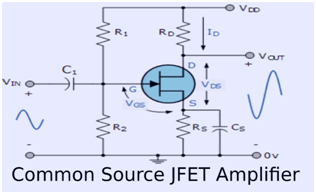The circuit of a common source N-channel JFET amplifier. It is similar to a common emitter amplifier. Hence the resistor R1 and R2 (called a voltage divider) are used to bias the field-effected transistor. The capacitor (C1) and(C2) are used to couple the a.c input voltage source and the output voltage respectively, these are known as coupling capacitor.

The capacitor (cs) keeps the source of the FET effectively at a.c ground and is known as bypass capacitor.

This produces variations in the drain current. As the gate-to-source voltage increase. As a result of this , the voltage drop across the resistor (RD) also increase. This cause the drain voltage to decrease.

It means that the positive half cycle of the input voltage produces the negative half cycle of the output voltage . in other words, the output voltage (at the drain) is 180O out of phase with the input voltage (at the gate).

AMPLIFIER DISTORTION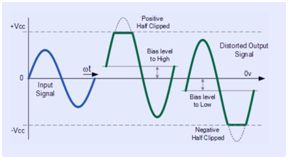Distortion in amplifier basically implies the variation in the waveform received at the output with respect to the applied input. Amplifier   distortion can take on many forms such as amplitude, frequency and phase distortion due to clipping. The unwanted alternation   generated during amplification is known as distortion.

Amplitude distortion: Amplitude distortion occurs due to following reasons-

(a) Amplitude Distortion Due to Incorrect Biasing
(b) Amplitude Distortion Due to Clipping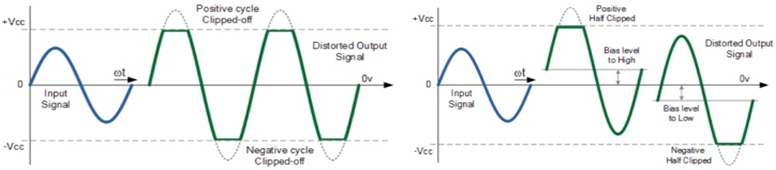(a) Amplitude Distortion Due to Incorrect Biasing                                    (b)Amplitude Distortion Due to Clipping

Frequency distortion: Frequency Distortion Due to Harmonics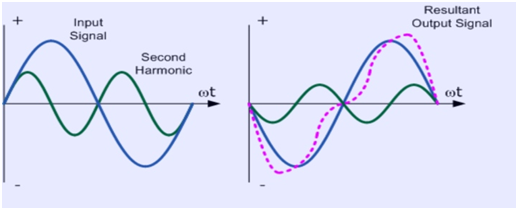(c) Phase distortion: Phase Distortion Due to Delay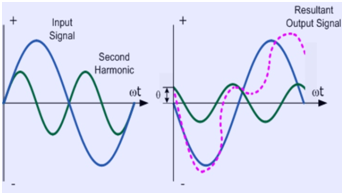CLASS A AMPLIFIER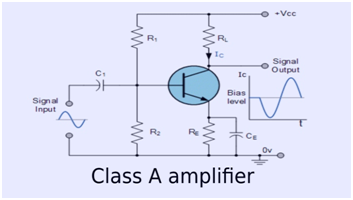• The power amplifier in which collector current flows during the whole cycle of the input signal are known as class A power amplifiers.
• In these amplifiers, biasing R1, R2, RE is so adjusted that the Q-point lies in middle of the load line and the collector current flows during the whole cycle of the input signal.
• As a result, the output shape is almost same the input, i.e., distortion is minimum. No part of the signal is cut off.
• However, these amplifier suffer from the disadvantage of very low efficiency which is about 35%.

Power amplifier efficiency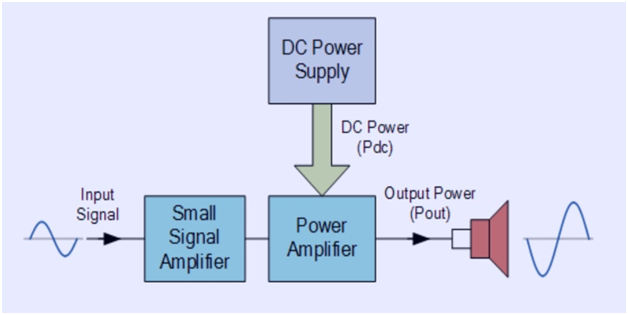Where:
η%  – is the efficiency of the amplifier.
Pout  – is the amplifiers output power delivered to the load.
Pdc  – is the DC power taken from the supply.

For a power amplifier it is very important that the amplifiers power supply is well designed to provide the maximum available continuous power to the output signal.

Transformer-Coupled Amplifier Circuit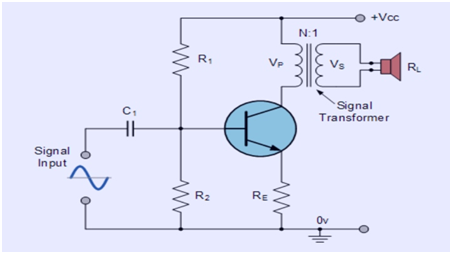Class A amplifier configuration can be calculated as follows.

The r.m.s. Collector voltage is given as:The r.m.s. Collector current is given as:The r.m.s. Power delivered to the load (Pac) is therefore given as:The average power drawn from the supply (Pdc) is given by:and therefore the efficiency of a Transformer-coupled Class A amplifier is given as:CLASS B AMPLIFIER: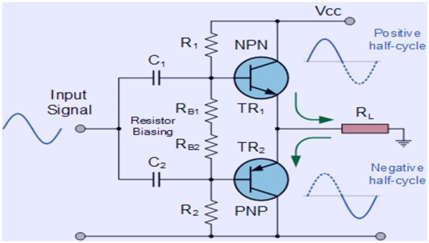The power amplifiers in which the operating point is so adjusted that the collector current, flows only during   the positive half cycle of the input signal are known as Class- B amplifiers.
For class-B operation of the amplifier, the biasing circuit is so adjusted that the operating point Q lies at the   collector cut-off voltage i.e., zero collector current IC =0.
During positive half-cycle of the signal, the input circuit is forward biased and hence collector current flows.   But, during negative half cycle of the signal, the input circuit is reverse biased and hence no collector current   flows.
In class-B amplifier, since the negative half-cycle of the signal is cut-off, it suffers from a severe distortion.   However, the points in favour of this amplifier are:
1. Higher power output
2. Higher collector efficiency (50 to 60%)
Such amplifier are mostly used for power amplification in push-pull arrangement.

CROSSOVER DISTORTION IN AMPLIFIERS